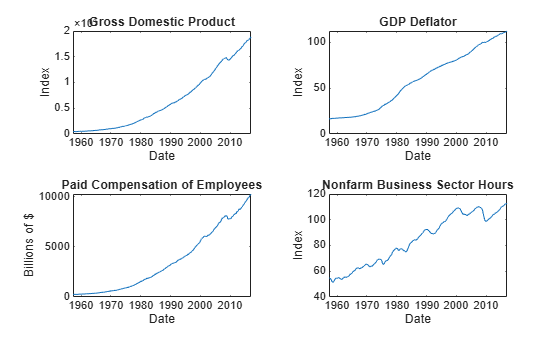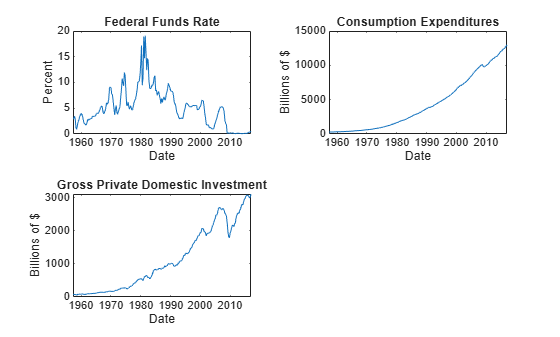# summarize

Display estimation results of vector error-correction (VEC) model

## Syntax

``summarize(Mdl)``
``results = summarize(Mdl)``

## Description

example

````summarize(Mdl)` displays a summary of the VEC(p – 1) model `Mdl`. If `Mdl` is an estimated VEC model returned by `estimate`, then `summarize` prints estimation results to the MATLAB® Command Window. The display includes an estimation summary and a table of parameter estimates with corresponding standard errors, t statistics, and p-values. The estimation summary includes fit statistics, such as the Akaike Information Criterion (AIC), and the estimated innovations covariance and correlation matrices.If `Mdl` is an unestimated VEC model returned by `vecm`, then `summarize` prints the standard object display (the same display that `vecm` prints during model creation). ```

example

````results = summarize(Mdl)` returns one of the following variables and does not print to the Command Window. If `Mdl` is an estimated VEC model, then `results` is a structure containing estimation results.If `Mdl` is an unestimated VEC model, then `results` is a `vecm` model object that is equal to `Mdl`. ```

## Examples

collapse all

Consider a VEC model for the following seven macroeconomic series, and then fit the model to the data.

• Gross domestic product (GDP)

• GDP implicit price deflator

• Paid compensation of employees

• Nonfarm business sector hours of all persons

• Effective federal funds rate

• Personal consumption expenditures

• Gross private domestic investment

Suppose that a cointegrating rank of 4 and one short-run term are appropriate, that is, consider a VEC(1) model.

Load the `Data_USEconVECModel` data set.

`load Data_USEconVECModel`

For more information on the data set and variables, enter `Description` at the command line.

Determine whether the data needs to be preprocessed by plotting the series on separate plots.

```figure; subplot(2,2,1) plot(FRED.Time,FRED.GDP); title('Gross Domestic Product'); ylabel('Index'); xlabel('Date'); subplot(2,2,2) plot(FRED.Time,FRED.GDPDEF); title('GDP Deflator'); ylabel('Index'); xlabel('Date'); subplot(2,2,3) plot(FRED.Time,FRED.COE); title('Paid Compensation of Employees'); ylabel('Billions of \$'); xlabel('Date'); subplot(2,2,4) plot(FRED.Time,FRED.HOANBS); title('Nonfarm Business Sector Hours'); ylabel('Index'); xlabel('Date');``````figure; subplot(2,2,1) plot(FRED.Time,FRED.FEDFUNDS); title('Federal Funds Rate'); ylabel('Percent'); xlabel('Date'); subplot(2,2,2) plot(FRED.Time,FRED.PCEC); title('Consumption Expenditures'); ylabel('Billions of \$'); xlabel('Date'); subplot(2,2,3) plot(FRED.Time,FRED.GPDI); title('Gross Private Domestic Investment'); ylabel('Billions of \$'); xlabel('Date');```Stabilize all series, except the federal funds rate, by applying the log transform. Scale the resulting series by 100 so that all series are on the same scale.

```FRED.GDP = 100*log(FRED.GDP); FRED.GDPDEF = 100*log(FRED.GDPDEF); FRED.COE = 100*log(FRED.COE); FRED.HOANBS = 100*log(FRED.HOANBS); FRED.PCEC = 100*log(FRED.PCEC); FRED.GPDI = 100*log(FRED.GPDI);```

Create a VEC(1) model using the shorthand syntax. Specify the variable names.

```Mdl = vecm(7,4,1); Mdl.SeriesNames = FRED.Properties.VariableNames```
```Mdl = vecm with properties: Description: "7-Dimensional Rank = 4 VEC(1) Model with Linear Time Trend" SeriesNames: "GDP" "GDPDEF" "COE" ... and 4 more NumSeries: 7 Rank: 4 P: 2 Constant: [7×1 vector of NaNs] Adjustment: [7×4 matrix of NaNs] Cointegration: [7×4 matrix of NaNs] Impact: [7×7 matrix of NaNs] CointegrationConstant: [4×1 vector of NaNs] CointegrationTrend: [4×1 vector of NaNs] ShortRun: {7×7 matrix of NaNs} at lag  Trend: [7×1 vector of NaNs] Beta: [7×0 matrix] Covariance: [7×7 matrix of NaNs] ```

`Mdl` is a `vecm` model object. All properties containing `NaN` values correspond to parameters to be estimated given data.

Estimate the model using the entire data set and the default options.

`EstMdl = estimate(Mdl,FRED.Variables)`
```EstMdl = vecm with properties: Description: "7-Dimensional Rank = 4 VEC(1) Model" SeriesNames: "GDP" "GDPDEF" "COE" ... and 4 more NumSeries: 7 Rank: 4 P: 2 Constant: [14.1329 8.77841 -7.20359 ... and 4 more]' Adjustment: [7×4 matrix] Cointegration: [7×4 matrix] Impact: [7×7 matrix] CointegrationConstant: [-28.6082 109.555 -77.0912 ... and 1 more]' CointegrationTrend: [4×1 vector of zeros] ShortRun: {7×7 matrix} at lag  Trend: [7×1 vector of zeros] Beta: [7×0 matrix] Covariance: [7×7 matrix] ```

`EstMdl` is an estimated `vecm` model object. It is fully specified because all parameters have known values. By default, `estimate` imposes the constraints of the H1 Johansen VEC model form by removing the cointegrating trend and linear trend terms from the model. Parameter exclusion from estimation is equivalent to imposing equality constraints to zero.

Display a short summary from the estimation.

`results = summarize(EstMdl)`
```results = struct with fields: Description: "7-Dimensional Rank = 4 VEC(1) Model" Model: "H1" SampleSize: 238 NumEstimatedParameters: 112 LogLikelihood: -1.4939e+03 AIC: 3.2118e+03 BIC: 3.6007e+03 Table: [133x4 table] Covariance: [7x7 double] Correlation: [7x7 double] ```

The `Table` field of `results` is a table of parameter estimates and corresponding statistics.

Consider the model and data in Estimate VEC Model and these four alternative VEC models: VEC(0), VEC(1), VEC(3), and VEC(7). Using historical data, estimate each of the four models, and then compare the model fits using the resulting Bayesian Information Criterion (BIC).

Load the `Data_USEconVECModel` data set and preprocess the data.

```load Data_USEconVECModel FRED.GDP = 100*log(FRED.GDP); FRED.GDPDEF = 100*log(FRED.GDPDEF); FRED.COE = 100*log(FRED.COE); FRED.HOANBS = 100*log(FRED.HOANBS); FRED.PCEC = 100*log(FRED.PCEC); FRED.GPDI = 100*log(FRED.GPDI);```

Within a loop:

• Create a VEC model using the shorthand syntax.

• Estimate the VEC Model. Reserve the maximum value of p as presample observations.

• Store the estimation results.

```numlags = [0 1 3 7]; p = numlags + 1; Y0 = FRED{1:max(p),:}; Y = FRED{((max(p) + 1):end),:}; for j = 1:numel(p) Mdl = vecm(7,4,numlags(j)); EstMdl = estimate(Mdl,Y,'Y0',Y); results(j) = summarize(EstMdl); end```

`results` is a 4-by-1 structure array containing the estimation results of each model.

Extract the BIC from each set of results.

`BIC = [results.BIC]`
```BIC = 1×4 103 × 5.3948 5.4372 5.8254 6.5536 ```

The model corresponding to the lowest BIC has the best fit among the models considered. Therefore, the VEC(0) model is the best fitting model.

## Input Arguments

collapse all

VEC model, specified as a `vecm` model object returned by `estimate` or `vecm`.

## Output Arguments

collapse all

Model summary, returned as a structure array or a `vecm` model object.

• If `Mdl` is an estimated VEC model, then `results` is a structure array containing the fields in this table.

FieldDescription
`Description`Model summary description (string)
`Model`Johansen model of deterministic terms (`"H2"`, `"H1*"`, `"H1"`, `"H*"`, `"H"`) 
`SampleSize`Effective sample size (numeric scalar)
`NumEstimatedParameters`Number of estimated parameters (numeric scalar)
`LogLikelihood`Optimized loglikelihood value (numeric scalar)
`AIC`Akaike Information Criterion (numeric scalar)
`BIC`Bayesian Information Criterion (numeric scalar)
`Table`Parameter estimates with corresponding standard errors, t statistics (estimate divided by standard error), and p-values (assuming normality); a table with rows corresponding to model parameters
`Covariance`Estimated residual covariance matrix (the maximum likelihood estimate), an `Mdl.NumSeries`-by-`Mdl.NumSeries` numeric matrix with rows and columns corresponding to the innovations in the response equations ordered by the columns of `Y`
`Correlation`Estimated residual correlation matrix whose dimensions correspond to the dimensions of `Covariance`

`summarize` uses `mvregress` to implement multivariate normal, maximum likelihood estimation. For more details on estimates and standard errors, see Estimation of Multivariate Regression Models (Statistics and Machine Learning Toolbox).

• If `Mdl` is an unestimated VEC model, then `results` is a `vecm` model object that is equal to `Mdl`.

 Johansen, S. Likelihood-Based Inference in Cointegrated Vector Autoregressive Models. Oxford: Oxford University Press, 1995.